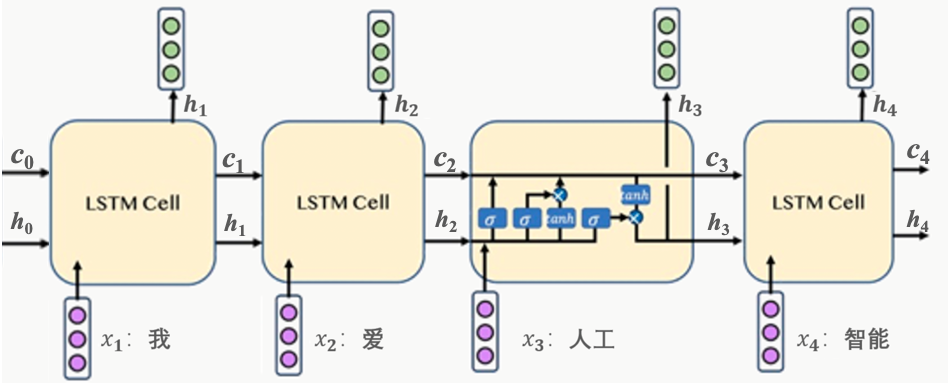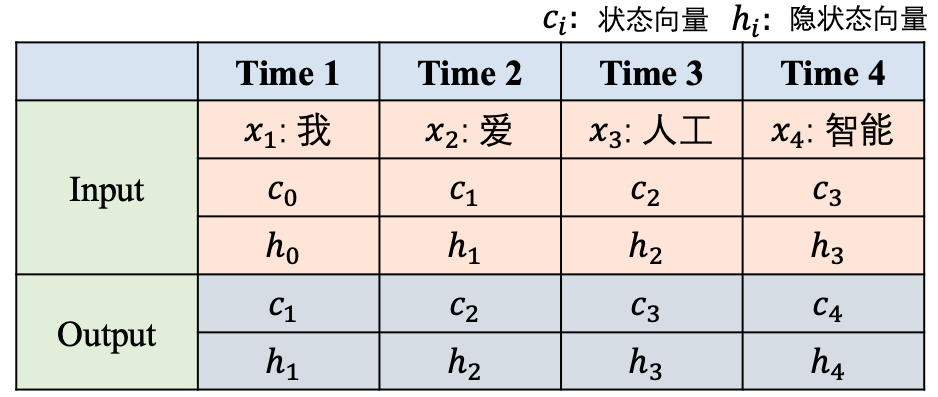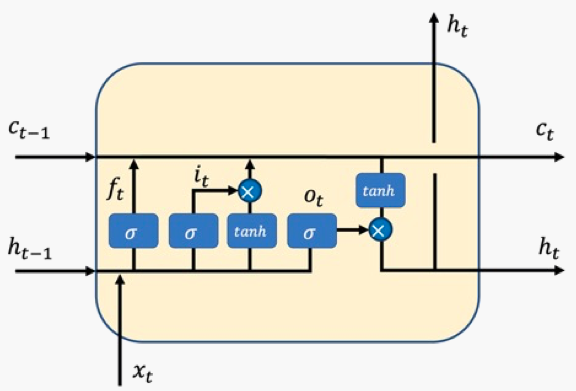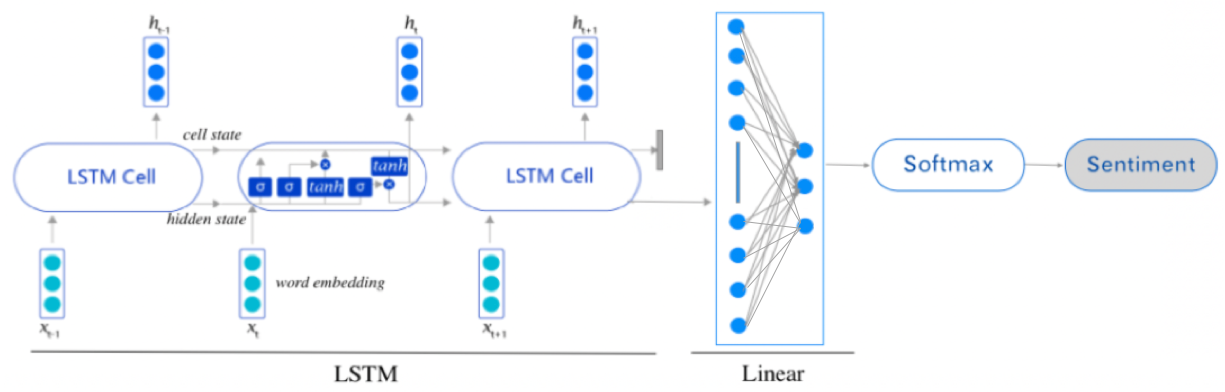# 长短时记忆网络 LSTM¶

## 2. LSTM是怎样工作的¶## 3. 从公式层面理解LSTM¶• 状态向量$$c_t$$：它控制着整个LSTM单元的状态或者记忆，它会根据每个时刻的输入进行更新，从而实时保持LSTM单元的记忆。

• 隐状态向量$$h_t$$：它是当前LSTM单元对外的输出状态，它是实际的工作状态向量，即一般会利用$$h_{t}$$来做一些具体的任务。

• 输入门$$i_t$$：控制当前时刻的输入信息需要向状态向量$$c_t$$中注入哪些信息。举个例子，当输入信息是一些没有实际意义的词，比如”的”，可能模型不会让这些信息流入到状态向量中，从而保持模型的语义表达。

• 遗忘门$$f_t$$：控制前一时刻的状态向量$$c_{t-1}$$需要被屏蔽/遗忘哪些信息。举个例子**，昨天我去爬了长城，哦不对是前天**， 当模型看到”不对，是前天”的时候，可能就会忘记前边的”昨天”。

• 输出门$$o_t$$：控制当前时刻的状态向量$$c_t$$需要对外输出哪些信息，最终输出的信息即为$$h_t$$

\begin{split}\begin{align} i_t &= sigmoid(W_ix_t+U_ih_{t-1}+b_i) \\ f_t &= sigmoid(W_fx_t+U_fh_{t-1}+b_f) \\ o_t &= sigmoid(W_ox_t+U_oh_{t-1}+b_o) \end{align}\end{split}

$a_t = tanh(W_ax_t+U_ah_{t-1}+b_a)$

$c_t = f_t \cdot c_{t-1} + i_t \cdot a_t$

$h_t=o_t \cdot tanh(c_t)$

## 4. 使用LSTM设计情感分析任务¶

### 4.1 情感分析是什么¶### 4.2 使用LSTM进行文本分类建模¶